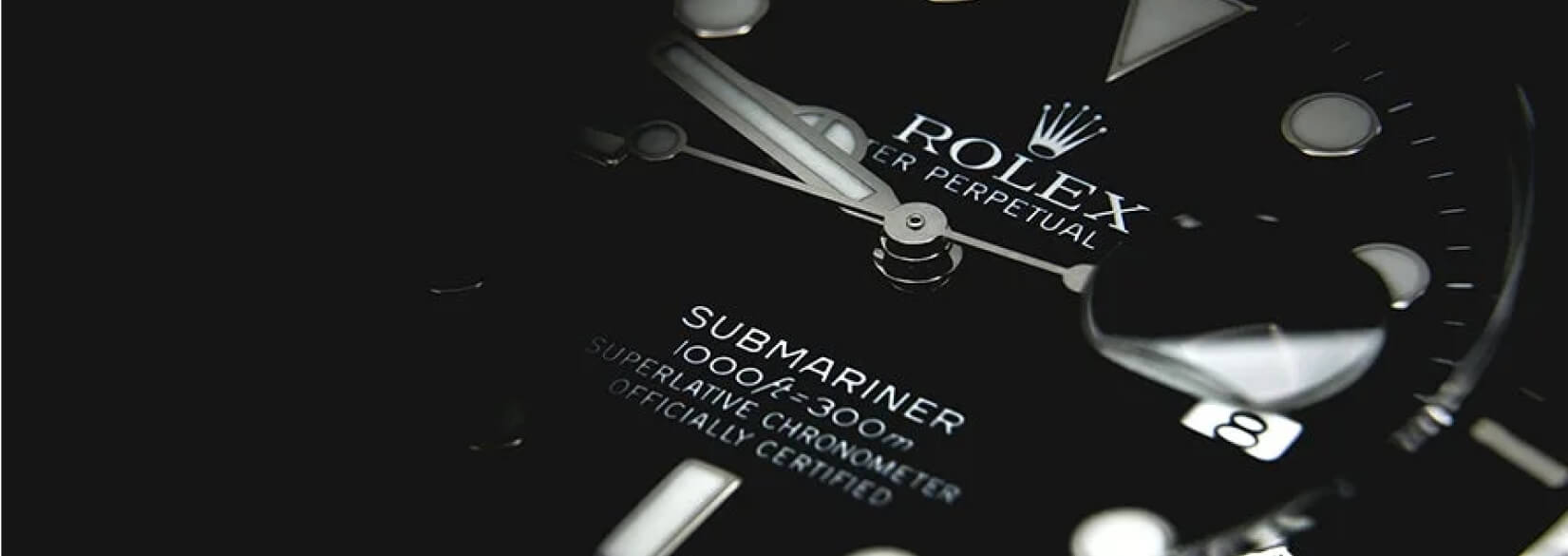# Case Study

## Kv & CoPriceless Timepieces Within Your Reach. Buy & Sell Your Designer Watches At KV&Co. Houston’s Luxury Timepieces Expert.## Problem

Here is where you add the problems. Here is where you add the problems. Here is where you add the problems. Here is where you add the problems. Here is where you add the problems. Here is where you add the problems. Here is where you add the problems.## Solution

Here is where you add the problems. Here is where you add the problems. Here is where you add the problems. Here is where you add the problems. Here is where you add the problems. Here is where you add the problems. Here is where you add the problems.## Outcome

Here is where you add the problems. Here is where you add the problems. Here is where you add the problems. Here is where you add the problems. Here is where you add the problems. Here is where you add the problems. Here is where you add the problems.## Website Design and Development

You can add the contents for this project here.

### Logo

You can add the contents for this project here. You can add the contents for this project here. You can add the contents for this project here. You can add the contents for this project here.

You can add the contents for this project here. You can add the contents for this project here. You can add the contents for this project here. You can add the contents for this project here.## Graphics

You can add the contents for this project here. You can add the contents for this project here: